## Python 内建数据类型

Posted on Jun 9, 2020# 数据类型概述

Python中包含了大量的数据类型（其他编程语言，例如Java，C++也是一样）。但其中有一些最为大家所频繁使用的就是内建数据类型。正如其名称所示，这些类型是内置在语言中的，不需要额外的库或者依赖。在满足要求的情况下，使用内建类型是比较好的选择。

• 可变类型：列表，字典，集合
• 不可变类型：数字，字符串，元祖

• (...)：元组
• [...]：列表
• {...}：字典，集合

# 数值类型

Python中的数值类型并非一种类型，而是一系列类型，它们如下表所示：

• 复数带有实部和虚部两个部分，虚部的表示是数字和j或者J作为后缀。例如：2 + 1j
• 小数是精度固定的浮点数，小数通过字符串来初始化，例如：Decimal('0.00001')
• 分数的实现保留了分子和分母两个部分。这样做的好处是避免了浮点运算的不精确性，坏处是性能较差（相对于浮点数）。

## 数值字面值

from decimal import Decimal
from fractions import Fraction

int_number = 1234
float_number = 0.1234
bool_number = True
complex_number = 3 + 4j
decimal_number = Decimal('0.1') + Decimal('0.2')
fraction_numer = Fraction(1, 3)

print(int_number, type(int_number))
print(float_number, type(float_number))
print(bool_number, type(bool_number))
print(complex_number, type(complex_number))
print(decimal_number, type(decimal_number))
print(fraction_numer, type(fraction_numer))


(1234, <type 'int'>)
(0.1234, <type 'float'>)
(True, <type 'bool'>)
((3+4j), <type 'complex'>)
(Decimal('0.3'), <class 'decimal.Decimal'>)
(Fraction(1, 3), <class 'fractions.Fraction'>)


## 进制与转换

• 0b或者0B作为前缀表示二进制，后面允许的数字是：0，1
• 0o或者0O作为前缀表示八进制，后面允许的数字是：0-7
• 0x或者0X作为前缀表示十六进制，后面允许的数字是：0-9和a-f或A-F

a = 0b111
b = 0o127
c = 0xAb

print('a =', a, 'bin:', bin(a), 'oct:', oct(a), 'hex:', hex(a))
print('b =', b, 'bin:', bin(b), 'oct:', oct(b), 'hex:', hex(b))
print('c =', c, 'bin:', bin(c), 'oct:', oct(c), 'hex:', hex(c))


a = 7 bin: 0b111 oct: 0o7 hex: 0x7
b = 87 bin: 0b1010111 oct: 0o127 hex: 0x57
c = 171 bin: 0b10101011 oct: 0o253 hex: 0xab


## 运算

x if y else z 三元选择表达式
x or y 逻辑或
x and y 逻辑与
not x 逻辑非
x in y, x not in y 成员关系，是否存在
x is y, x is not y 同一性测试，是否相同
x < y, x <= y, x > y, x >= y 大小比较
x == y, x != y 值等价性判断
x | y 按位或，集合并集
x ^ y 按位异或，集合对称差集
x & y 按位与，集合交集
~x 按位非
x « y, x » y 左移和右移操作
x + y, x - y, x * y 加法，减法，乘法
x % y 求余数
x / y, x // y 真除法和向下取整除法
-x, +x 取负，取正
x ** y 幂运算

## 链式比较

a = 55
b = 101

print('1 <= a <= 100: ', 1 <= a <= 100)
print('1 <= b <= 100: ', 1 <= b <= 100)


1 <= a <= 100:  True
1 <= b <= 100:  False


## 除法

a = 100
b = 3

print(a, '/', b, '=', a / b)
print(a, '//', b, '=', a // b)


100 / 3 = 33.333333333333336
100 // 3 = 33


# 字符串

Python没有单个字符的数据类型，只有包含一系列字符的字符串类型。

## 字符串字面值

• 单引号：'simple string'
• 双引号："It's a tiger"
• 三引号：'''...content...'''"""...content..."""
• 原始字符串格式：r"C:\folder\file.txt"
• 字节字面值
• Unicode字面值

a = 'string content'
b = "string content"
c = 'He says "hello"'
d = "It's a nice day."

print(a == b)
print(c)
print(d)


True
He says "hello"
It's a nice day.


'''
STAR
WARS

A long time ago in a galaxy far,
far away...
'''


## 字符串的方法

str.capitalize()
str.casefold()
str.center(width[, fillchar])
str.count(sub[, start[, end]])
str.encode(encoding="utf-8", errors="strict")
str.endswith(suffix[, start[, end]])
str.expandtabs(tabsize=8)
str.find(sub[, start[, end]])
str.format(*args, **kwargs)
str.format_map(mapping)
str.index(sub[, start[, end]])
str.isalnum()
str.isalpha()
str.isascii()
str.isdecimal()
str.isdigit()
str.isidentifier()
str.islower()
str.isnumeric()
str.isprintable()
str.isspace()
str.istitle()
str.isupper()
str.join(iterable)
str.ljust(width[, fillchar])
str.lower()
str.lstrip([chars])
static str.maketrans(x[, y[, z]])
str.partition(sep)
str.replace(old, new[, count])
str.rfind(sub[, start[, end]])
str.rindex(sub[, start[, end]])
str.rjust(width[, fillchar])
str.rpartition(sep)
str.rsplit(sep=None, maxsplit=-1)
str.rstrip([chars])
str.split(sep=None, maxsplit=-1)
str.splitlines([keepends])
str.startswith(prefix[, start[, end]])
str.strip([chars])
str.swapcase()
str.title()
str.translate(table)
str.upper()
str.zfill(width)


## 格式化

Python中下面两种格式化写法都很常用：

• %形式：'...%s... % (values)
• format方法：'...{}...'.format(values)

template = 'Star.Wars.Episode.%d.%s.%d'
year = 1999
print(template % (1, 'The.Phantom.Menace', year))


Star.Wars.Episode.1.The.Phantom.Menace.1999


template2 = 'Star.Wars.Episode.%(number)d.%(title)s.%(year)d'
content = {'number': 2, 'title': 'Attack.of.the.Clones', 'year': 2002}
print(template2 % content)


Star.Wars.Episode.2.Attack.of.the.Clones.2002


template3 = 'Star.Wars.Episode.{}.{}.{}'
print(template3.format(3, 'Revenge.of.the.Sith', 2005))


Star.Wars.Episode.3.Revenge.of.the.Sith.2005


template4 = 'Star.Wars.Episode.{2}.{1}.{0}'
print(template4.format(1977, 'A.New.Hope', 4))


Star.Wars.Episode.4.A.New.Hope.1977


template5 = 'Star.Wars.Episode.{number}.{title}.{year}'
print(template5.format(number=5, title='The.Empire.Strikes.Back', year=1980))


Star.Wars.Episode.5.The.Empire.Strikes.Back.1980


# 集合

Python中的set类实现了数学中集合的概念。

set_a = set([1, 2, 3, 4, 5])
set_b = {3, 4, 5, 6, 7}


set_a = set([1, 2, 3, 4, 5])
set_b = {3, 4, 5, 6, 7}

print('set a:', set_a)
print('set b:', set_b)
print('----------------------')
print('a - b:', set_a - set_b)
print('a ^ b:', set_a ^ set_b)
print('a & b:', set_a & set_b)
print('a | b:', set_a | set_b)


set a: {1, 2, 3, 4, 5}
set b: {3, 4, 5, 6, 7}
----------------------
a - b: {1, 2}
a ^ b: {1, 2, 6, 7}
a & b: {3, 4, 5}
a | b: {1, 2, 3, 4, 5, 6, 7}


# 列表

list_a = [1, 2, 3, 4, 5]
list_b = list('a', 'b', 'c', 'd', 'e')


L1 + L2 拼接
L * 3 重复
L.append(1) 尾部添加
L.extend([2, 3, 4]) 尾部扩展
L.insert(i, X) 为位置i插入值X
L.index(X) 返回X的索引位置
L.count(X) 统计X出现的次数
L.sort() 排序
L.reverse() 反转
L.copy() 复制
L.clear() 清除所有元素
L.pop(i) 删除i处元素，并返回
L.remove(X) 删除元素X
del L[i] 删除i处元素
del L[i:j] 删除i到j处元素
L[i:j] = [] 删除i到j处元素
L[i] = 3 索引赋值

list_a = [1, 2, 3, 4, 5]
print('a:', list_a)

list_a.append(1);
print('after append 1, a:', list_a)

list_a.extend([1,2,3])
print('after extend [1, 2, 3], a:', list_a)

print("index of '1' in a:", list_a.index(1))

list_a.sort()
print('after sort, a: ', list_a)

list_a.reverse()
print('after reverse, a:', list_a)

list_a.remove(1)
print('after remove(1), a:', list_a)

del list_a[1:3]
print('after del a[1:3], a:', list_a)

list_b = list('abcde')
print('b:', list_b)
list_c = list_a + list_b
print('a + b:', list_c)


a: [1, 2, 3, 4, 5]
after append 1, a: [1, 2, 3, 4, 5, 1]
after extend [1, 2, 3], a: [1, 2, 3, 4, 5, 1, 1, 2, 3]
index of '1' in a: 0
after sort, a:  [1, 1, 1, 2, 2, 3, 3, 4, 5]
after reverse, a: [5, 4, 3, 3, 2, 2, 1, 1, 1]
after remove(1), a: [5, 4, 3, 3, 2, 2, 1, 1]
after del a[1:3], a: [5, 3, 2, 2, 1, 1]
b: ['a', 'b', 'c', 'd', 'e']
a + b: [5, 3, 2, 2, 1, 1, 'a', 'b', 'c', 'd', 'e']


# 字典

dic.keys() 返回所有键
dic.values() 返回所有值
dic.items() 所有“键 + 值”元组
dic.copy() 复制
dic.clear() 清除所有条目
dic.get(key, default?) 通过键获取，如果不存在返回default值
dic.pop(key, default?) 通过键删除，如果不存在返回default值
dic.popitem() 删除并返回所有键值对
dic[key] = 42 通过键赋值
del D[key] 根据键删除条目

paul = dict(Name='Paul', Hobby=['Music', 'Photograph'], Job='Developer')
moira = dict(zip(['Name', 'Hobby', 'Job'], ['Moira', 'Movie', 'Student']))

family = {'People': [paul, moira], 'City': 'Nanjing'}

print('paul.keys:', paul.keys())
print('paul.values:', paul.values())
print('paul.City:', paul.get('City', 'Nanjing'))

print('Family, city:', family['City'])

for p in family['People']:
print('\t', p)

print("Moira's hobby is:", moira['Hobby'])

paul['Hobby'] = ['Rock', 'Computer']
print('paul:', paul)
del paul['Hobby']
print('paul:', paul)


paul.keys: dict_keys(['Name', 'Hobby', 'Job'])
paul.values: dict_values(['Paul', ['Music', 'Photograph'], 'Developer'])
paul.City: Nanjing
Family, city: Nanjing
{'Name': 'Paul', 'Hobby': ['Music', 'Photograph'], 'Job': 'Developer'}
{'Name': 'Moira', 'Hobby': 'Movie', 'Job': 'Student'}
Moira's hobby is: Movie
paul: {'Name': 'Paul', 'Hobby': ['Rock', 'Computer'], 'Job': 'Developer'}
paul: {'Name': 'Paul', 'Job': 'Developer'}


# 元组

t1 + t2 拼接
t * 3 重复
t.count(‘a’) 返回元素出现次数
t.index(‘a’) 返回第一次找到的元素下标

t1 = (1, '2', (3, 4))
t2 = tuple([1, '2', [3,4]])
t3 = t1 + t2

print('len(t3): ', len(t3))
print('t3.count(1): ', t3.count(1))
print("t3.index('2'): ", t3.index('2'))


len(t3):  6
t3.count(1):  2
t3.index('2'):  1


# 成员关系与迭代

• 通过in确认成员关系。注意：对于字典来说，in是指键所组成的集合。
• 通过for x in y来遍历其中的元素。
• 来遍历的时候，你可以使用表达式创建新的数据。
t = (1, '2', 3.0)
l = [1, 2, 3.0]
s = {1, '2', 3.0}
d = {'Name': 'Paul', 'Hobby': ['Music', 'Photograph']}
str = 'Attack.of.the.Clones'

print('1 in', t, ':', 1 in t)
print('1 in', l, ':', 1 in l)
print('1 in', s, ':', 1 in s)
print('"Name" in', d, ':', 'Name' in d)
print('"Attack" in', str, ':', "Attack" in str)

print('\nelements in list:')
for x in l:
print('\t', x)
print('\n')

new_l = [x ** 2 for x in l]
print('new_l:', new_l)


1 in (1, '2', 3.0) : True
1 in [1, 2, 3.0] : True
1 in {1, 3.0, '2'} : True
"Name" in {'Name': 'Paul', 'Hobby': ['Music', 'Photograph']} : True
"Attack" in Attack.of.the.Clones : True

elements in list:
1
2
3.0

new_l: [1, 4, 9.0]


# 索引和分片

• l[:]是指获取集合l中的所有元素
• l[n:m]是指获取[n, m)范围内的元素
• l[:9]是指获取[0,9)范围内的元素
• l[n:]是指获取第n个开始到末尾的元素
• l[:-n]是指获取[0, len(l)-n)范围内的元素
• l[n:m:d]是指获取[n, m)范围内的元素，但是每隔d次取一个元素

str = "Star.Wars.Episode.4.A.New.Hope.1977"

print('str[:]:', str[:])
print('str[5:9]:', str[5:9])
print('str[:9]:', str[:9])
print('str[5:]:', str[5:])
print('str[:-8]:', str[:-16])
print('str[::2]', str[::2])


str[:]: Star.Wars.Episode.4.A.New.Hope.1977
str[5:9]: Wars
str[:9]: Star.Wars
str[5:]: Wars.Episode.4.A.New.Hope.1977
str[:-8]: Star.Wars.Episode.4
str[::2] Sa.asEioe4ANwHp.97


# 变量与对象

Python与其他语言有一个很大的区别是：Python中的变量不需要声明类型。实际上，变量本身并不存在类型，类型是对象才有的属性。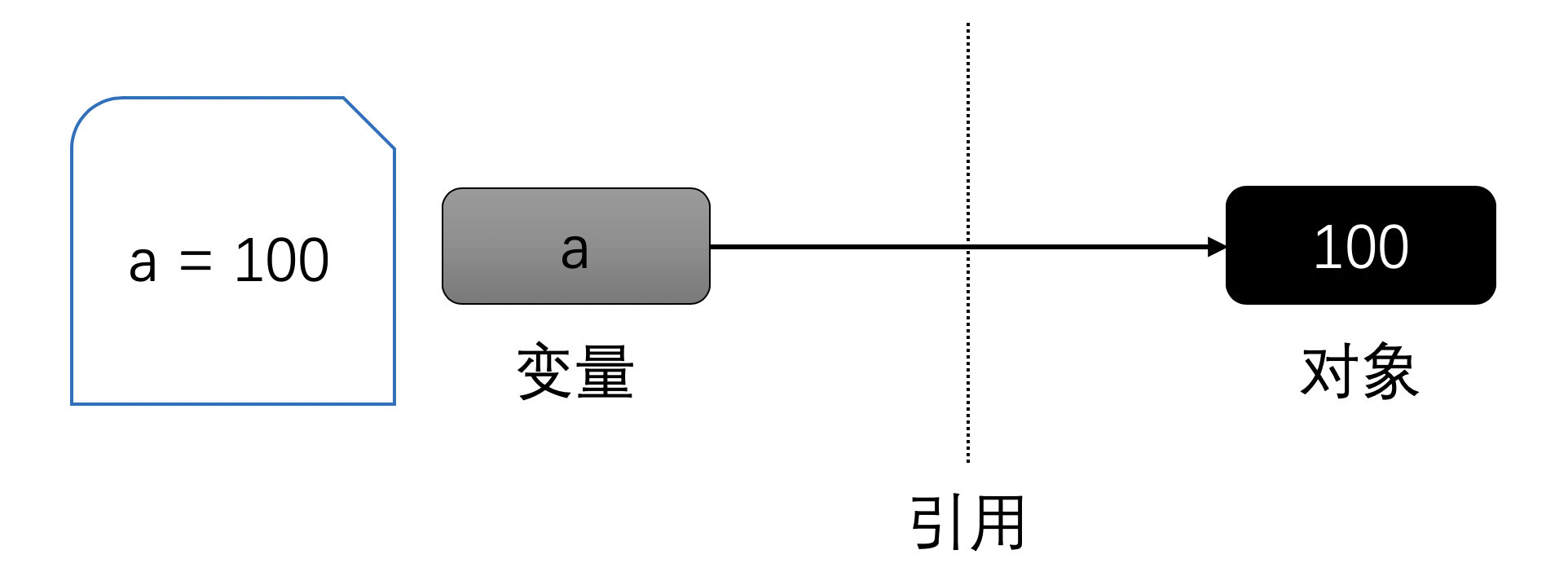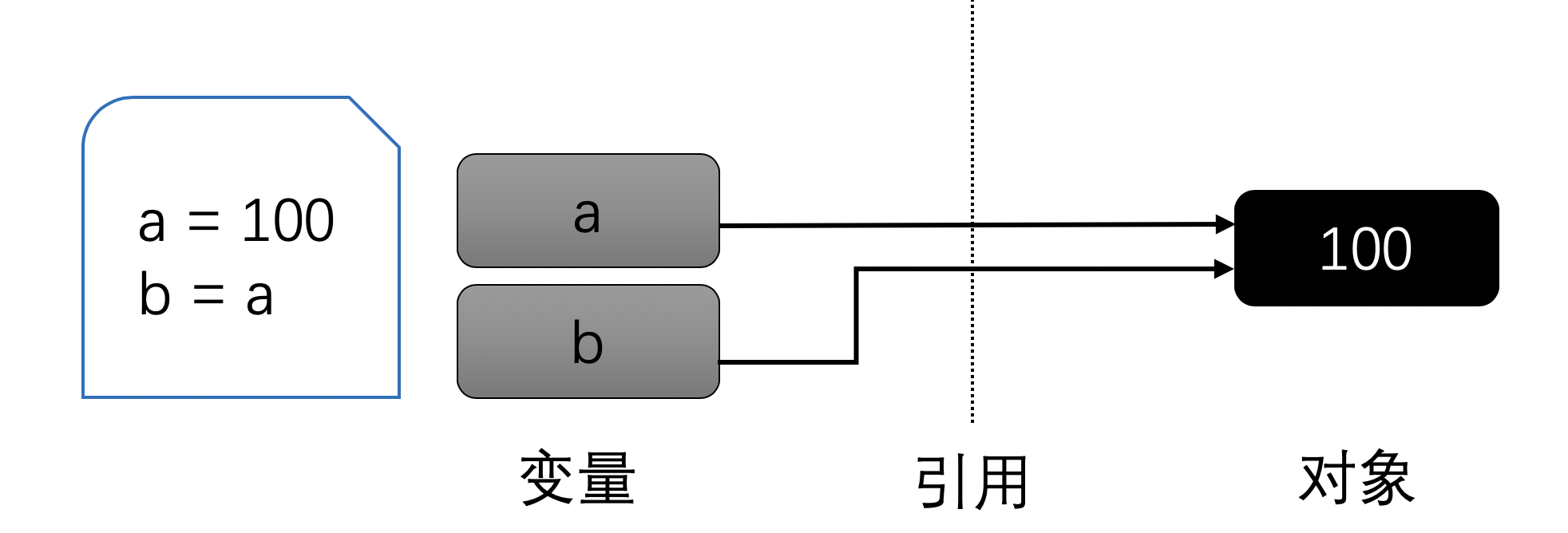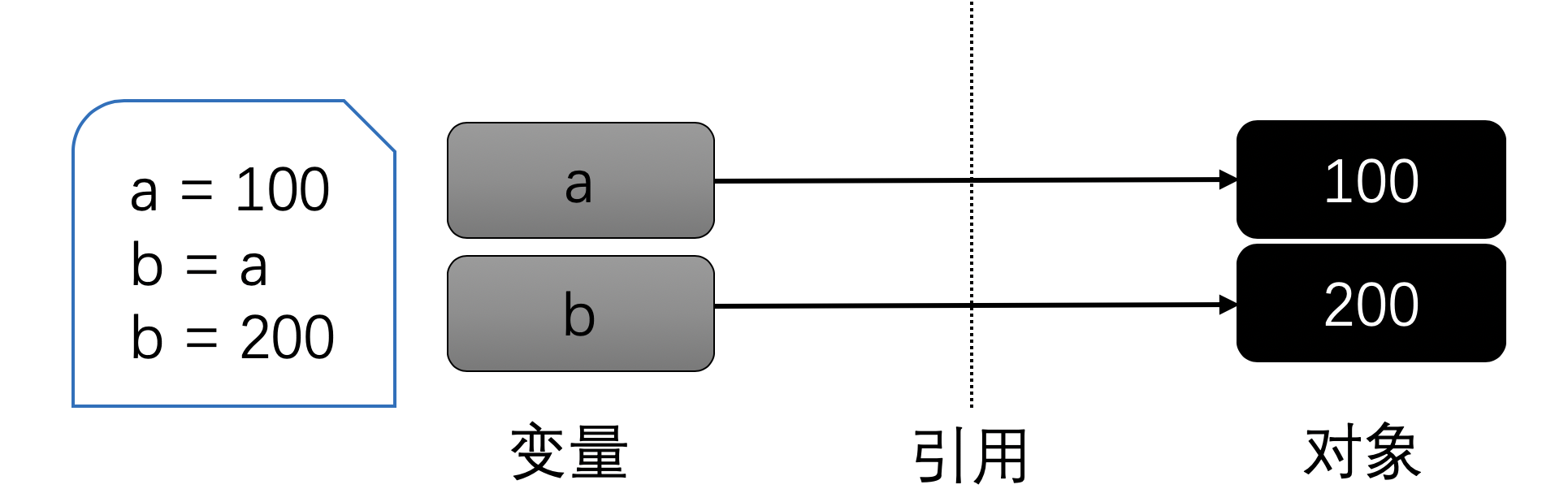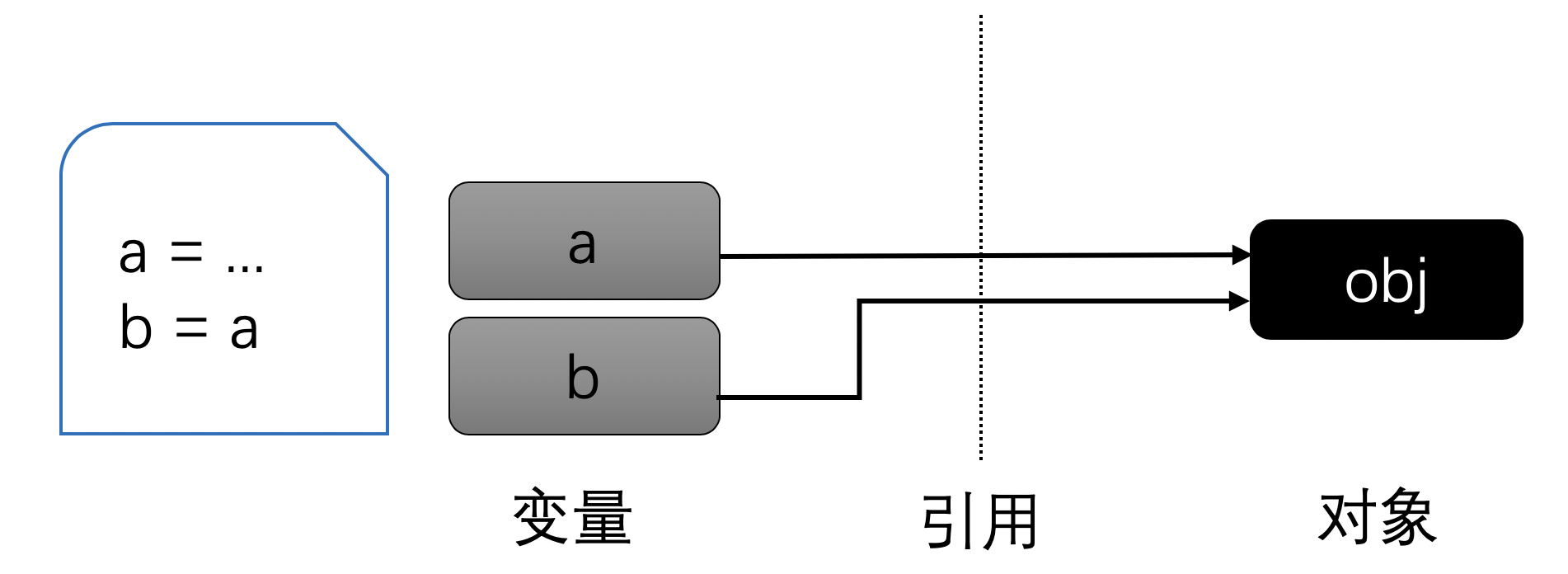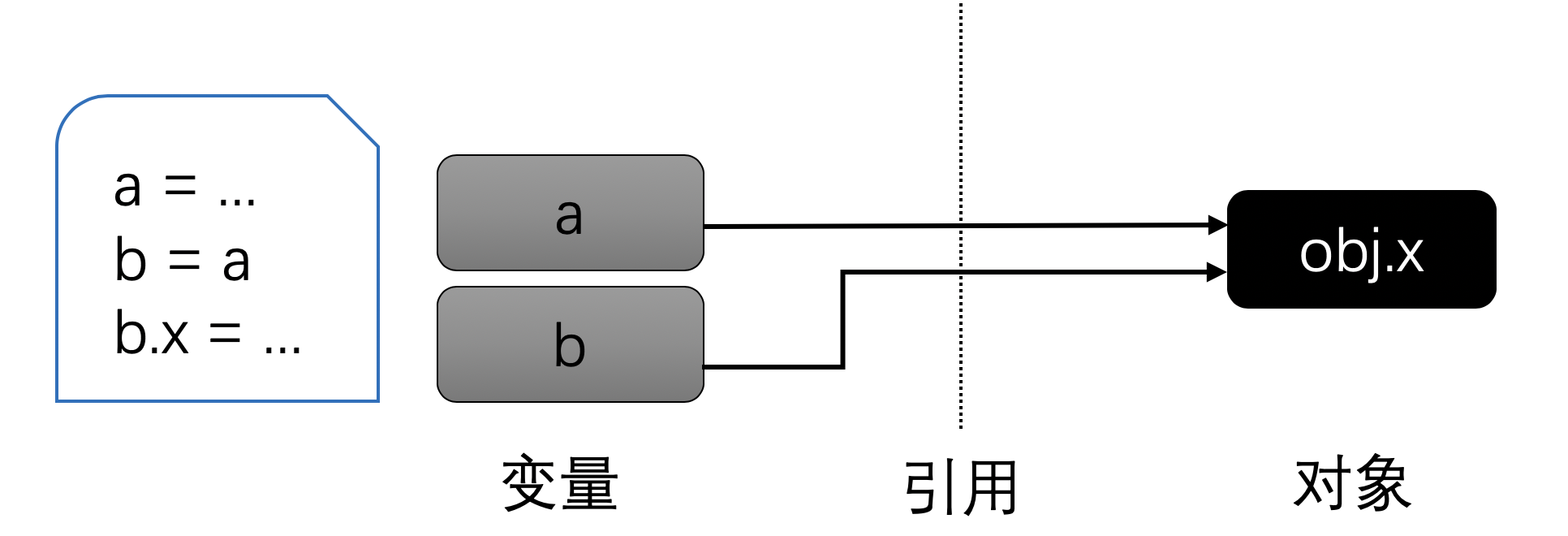## ==和is

• ==是判断两个对象的值是否相等
• is是判断两个变量是否指向同一个对象

a = 100
b = a;
print('a==b:', a==b)
print('a is b:', a is b)


b = 100
print('a==b:', a==b)
print('a is b:', a is b)


a = [1, 2, 3]
b = a
print('a==b:', a==b)
print('a is b:', a is b)

a = 100
print('a:', a)
print('b:', b)

b = [1, 2, 100]
print('a==b:', a==b)
print('a is b:', a is b)


a==b: True
a is b: True
a: [1, 2, 100]
b: [1, 2, 100]
a==b: True
a is b: False


Contents